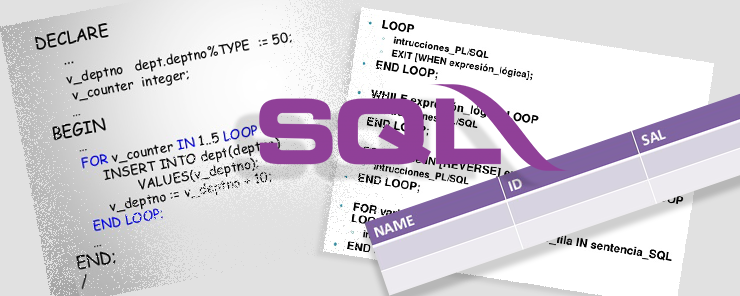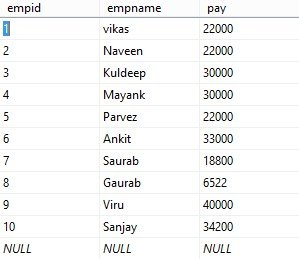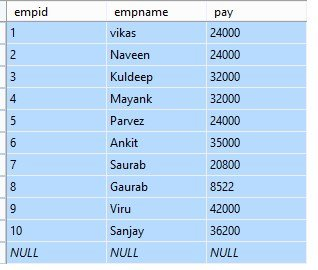# Learn While loop in SQL

1
3742The looping statement repeatedly executes command in its block until the specified condition become true or it terminates by break keyword.
SQL extensively use While loop statement to iterate the PL/SQL statement because this is the only looping statement support by SQL.
IN this article we are going to learn to how to use of While Loop, Break Keyword, and Continue keyword in sql.

While Loop: The statement in code block execute repeatedly until condition specified in Boolean expression become satisfied or it terminate by break keyword. Here is the basic syntax of while loop.

```WHILE Boolean_expression
begin
{code_block | BREAK | CONTINUE }
end
```

Example: This example show Multiplication of tables. I will increment the count from 1 to 10 and then do multiplication of count with specific number. Let’s say table of 2

```DECLARE
@tableof tinyint =2,
@count tinyint

SELECT @count = 1
WHILE @count <= 10
BEGIN
print  cast(@tableof as varchar(2))+ ' * ' +  cast(@count as varchar(10)) + ' = '  +  cast( @tableof * @count as varchar(10))
set @count = @count +1
END
```

The above looping statement gives below results

```2 * 1 = 2
2 * 2 = 4
2 * 3 = 6
2 * 4 = 8
2 * 5 = 10
2 * 6 = 12
2 * 7 = 14
2 * 8 = 16
2 * 9 = 18
2 * 10 = 20
```

Break-Keyword: It stops the execution of while loop or it terminates the execution process of while loop

Example: in this example I am terminating the execution of statement when count values reach to 5

```DECLARE
@tableof tinyint =2,
@count tinyint

SELECT @count = 1
WHILE @count <= 10
BEGIN
print  '2  * ' + cast (@count as varchar(2))  +' = ' +  cast( @tableof * @count as varchar(10))
set @count = @count +1

if @count =5
break;   -- the loop will terminate the execution when @count reach to 5
END
```

According the above looping statement it gives following result:

```2  * 1	= 2
2  * 2 = 4
2  * 3 = 6
2  * 4 = 8
```

Continue- keyword: It skips the execution of all the statement after its execution and start execution from first statement of while loop.

```DECLARE
@tableof tinyint =2,
@count tinyint

SELECT @count = 1
WHILE @count <= 10
BEGIN
print  '2  * ' + cast (@count as varchar(2))  +' = ' +  cast( @tableof * @count as varchar(10))
set @count = @count +1
continue     -- The control of execution move to first statement of loop when it reach to continue keyword
if @count =5 -- This statement will skip from execution and will never execute
break;
END
```

This will give following result after execution

```2  * 1 = 2
2  * 2 = 4
2  * 3 = 6
2  * 4 = 8
2  * 5 = 10
2  * 6 = 12
2  * 7 = 14
2  * 8 = 16
2  * 9 = 18
2  * 10 = 20```

Example : In this example I am going to update the salary table . I will update the basic pay to increment 2000 to each employee as per bonus
Let first look on the salary table:We will make loop to update basic pay as 2000 increment for each employee.

```declare @totalrecord int
declare @step int =1
select @totalrecord = count(*) from tblsalary

while @step <= @totalrecord
begin

update tblsalary set pay = pay +2000 where empid [email protected]

set @step [email protected] + 1
end
```

After execution of above looping statement , we can see that basic pay is hike with 2000 for each employeeHence, we have learn the While loop in SQL.

#### 1 COMMENT

1.sahil

Very informative blog.